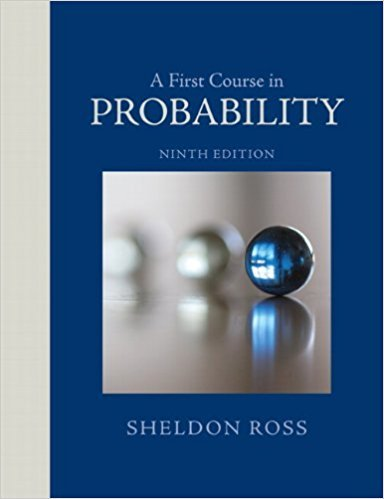×
Get Full Access to A First Course In Probability - 9 Edition - Chapter 3 - Problem 23ste
Get Full Access to A First Course In Probability - 9 Edition - Chapter 3 - Problem 23ste

×

# Let A and B be events having positive probability. StateISBN: 9780321794772 63

## Solution for problem 23STE Chapter 3

A First Course in Probability | 9th Edition

• Textbook Solutions
• 2901 Step-by-step solutions solved by professors and subject experts
• Get 24/7 help from StudySoup virtual teaching assistantsA First Course in Probability | 9th Edition

4 5 1 374 Reviews
31
0
Problem 23STE

Problem 23STE

Let A and B be events having positive probability. State whether each of the following statements is (i) necessarily true, (ii) necessarily false, or (iii) possibly true.

(a) If A and B are mutually exclusive, then they are independent.

(b) If A and B are independent, then they are mutually exclusive.

(c) P(A) = F(B) = .6, and A and B are mutually exclusive.

(d) P(A) = P(B) = .6, and A and B are independent.

Step-by-Step Solution:

Solution:

Step 1 of 5:

Let A and B are events with positive probability.

We have to find the given statements are (i) necessarily true, (ii) necessarily false, or (iii) possibly true.

1. If A and B are mutually exclusive, then they are independent.
2. If A and B are independent, then they are mutually exclusive.
3. P(A), PB) = 0.6, then A and B are mutually exclusive.
4. P(A), P(B) = 0.6, and A and B are independent.

Step 2 of 5

Step 3 of 5

##### ISBN: 9780321794772

Unlock Textbook Solution